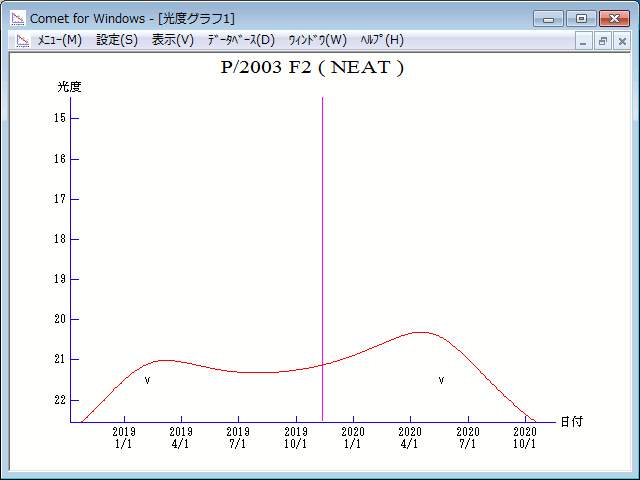# \$B%K!<%HWB@1(B

P/2003 F2 ( NEAT ) 2019###\$B50F;MWAG(B

```Perturbed ephemeris below is based on elements from MPC 50354.

Epoch = 2458800.50000 TT   T = 2458799.47896 TT
Peri. =  191.82521         a =     6.4839723 AU
Node  =  359.09123 J2000.0 e =     0.5419496
Incl. =   11.60599         q =     2.9699860 AU
```

###\$B8wEYJQ2=(B

```        m1 = 13.5 + 5 log\$B&\$(B + 10.0 log r
```##### \$B50F;MWAG\$O(BMinor Planet & Comet Ephemeris Service\$B\$G7W;;\$7\$?\$b\$N\$G\$9!#(B \$B8wEY%0%i%U\$O(BComet for Windows\$B\$G:n@.\$7\$?\$b\$N\$G\$9!#(B